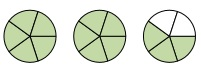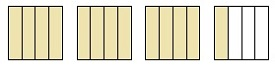# Writing a mixed number and an improper fraction for a shaded region

#### Complete Python Prime Pack

9 Courses     2 eBooks

#### Artificial Intelligence & Machine Learning Prime Pack

6 Courses     1 eBooks

#### Java Prime Pack

9 Courses     2 eBooks

In this lesson, we consider figures like circles, rectangular strips etc., some of which are shaded whole and some shaded fractionally. For combinations of whole and fractionally shaded regions, we write the corresponding mixed numbers and improper fractions that would represent them.

For example, if two whole shaded figures and a three-fifth fractionally shaded figure are given, we represent such a combination with mixed number $2\frac{4}{5}$ and improper fraction $\frac{14}{5}$.

Write a mixed number for the shaded region given below### Solution

Step 1:

There are two shaded whole circles and a three-fifth shaded circle.

Step 2:

$1 + 1 + \frac{3}{5} = 2\frac{3}{5}$

So, this combination of figures is represented by the mixed number $2\frac{3}{5}$.

Write an improper fraction for the shaded region given below### Solution

Step 1:

There are three shaded whole rectangular strips and a one-fourth shaded strip.

Step 2:

$1 + 1 + 1 + \frac{1}{4} = 3\frac{1}{4}$

So, this combination of figures is represented by the mixed number $3\frac{1}{4}$

Step 3:

$3\frac{1}{4}$ is written as an improper fraction using an algorithm as follows.

$3\frac{1}{4} = \frac{\left ( 3 \times 4 + 1 \right )}{4} = \frac{13}{4}$

So, the improper fraction representing the given shaded region is $\frac{13}{4}$.